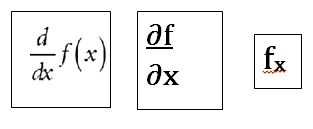# Solving Partial Derivative Equations

Lesson Transcript
Instructor: Tiffany Price

Tiffany has many years of classroom teaching experience and has a masters degree in Educational Leadership.

In this lesson, we'll introduce partial derivative equations and look into how they are solved. We'll explore several of the derivative rules and apply those rules to solve a few examples.

## Derivatives

Derivatives, in general, are important in calculus in that they allow us to see the value of a function at a particular point. Partial derivatives are very similar to solving total derivatives because the same rules apply to both. Total derivatives allow all of the variables in an equation to change, while partial derivatives only differentiate one variable at a time while the other variable(s) remain fixed.

An error occurred trying to load this video.

Try refreshing the page, or contact customer support.

Coming up next: Higher-Order Partial Derivatives Definition & Examples

### You're on a roll. Keep up the good work!

Replay
Your next lesson will play in 10 seconds
• 0:03 Derivatives
• 0:28 Rules and Notation
• 1:34 Partial Derivative Examples
• 4:28 Lesson Summary
Save Save

Want to watch this again later?

Timeline
Autoplay
Autoplay
Speed Speed

## Rules and Notation

The easiest way to solve both partial and total derivatives is to memorize the shortcut derivative rules or have a chart of the rules handy. A few of the rules for solving derivative equations are:

• The constant rule: the derivative of a constant is 0

• The line rule: the derivative of nx (a first order variable) is equal to its coefficient (n)

• The power rule: the derivative of xn (any variable to a power) is nx(n-1)

• Logarithms rule: the derivative of ln(x) is 1/x

• Chain rule: the derivative of more complicated functions is (derivative of the outside function leaving inside alone) x (derivative of inside function)

There are many more rules than these, but in this lesson, we will only look at these five. When solving partial derivatives, the variable that is not being differentiated is treated like a constant.

Also, be aware that there is no uniform symbol to represent differentiation. Here are a few notations you may come across when solving these functions:## Partial Derivative Examples

Let's solve a couple of equations:

Find the derivative of this equation with respect to x: f(x,y) = 6x - 9y³

df/dx (x,y) = 6x - 9

Because the derivative of 6x is 6, and - 9 is treated like a constant (whose derivative is 0), the answer is 6

df/dx (x,y) = 6 - 0 = 6

Let's look at the same equation and differentiate the y variable only.

df/dy (x,y) = 6x - 9

This time the derivative of 6x will be 0 because we're treating it as a constant. The derivative of -9 is - 27 using the power rule.

df/dy (x,y) = 0 - 9(3)y(3-1) = - 27

To unlock this lesson you must be a Study.com Member.

### Register to view this lesson

Are you a student or a teacher?